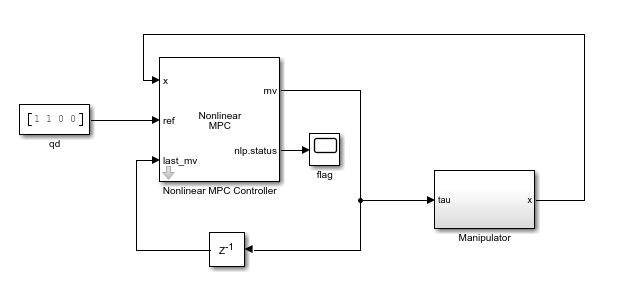# Control Robot Manipulator Using Passivity-Based Nonlinear MPC

This example shows how to design a passivity-based controller for a robot manipulator using nonlinear model predictive control (MPC).

### Overview

The dynamics for a two-link robot manipulator can be written as follows .

`$\mathit{H}\left(\mathit{q}\right)\stackrel{¨}{\mathit{q}}+\mathit{C}\left(\mathit{q},\stackrel{˙}{\mathit{q}}\right)\stackrel{˙}{\mathit{q}}+\mathit{G}\left(\mathit{q}\right)=\tau$`

Here, $\mathit{q}$, $\stackrel{˙}{\mathit{q}}$, and $\stackrel{¨}{\mathit{q}}$ are 2-by-1 vectors that represent the joint angles, velocities, and accelerations. The control input vector is the torque $\tau$.

• $\mathit{H}\left(\mathit{q}\right)$ is the manipulator inertia matrix.

• $\mathit{C}\left(\mathit{q},\stackrel{˙}{\mathit{q}}\right)$ is the Coriolis matrix.

• $\mathit{G}\left(\mathit{q}\right)$ is the gravity vector.

These robot dynamics are implemented in `manipulatorStateFcn.m`.

The control objective is to select torque $\tau$ such that the joint angles $\mathit{q}$ track a desired reference ${\mathit{q}}_{\mathit{d}}$. To enforce closed-loop stability, the controller includes a passivity constraint .

### Passivity Constraint

To define the passivity constraint, first define the tracking error vector as the difference between the joint angles and the desired reference angles.

`${\mathit{e}}_{\mathit{q}}=\mathit{q}-{\mathit{q}}_{\mathit{d}}$`

To achieve good tracking performance, define the storage function as $\mathit{V}=\frac{1}{2}\left({\stackrel{˙}{\mathit{q}}}^{\mathit{T}}\mathit{H}\left(\mathit{q}\right)\stackrel{˙}{\mathit{q}}+{\mathit{e}}_{\mathit{q}}^{\mathit{T}}{\mathrm{Ke}}_{\mathit{q}}\right)$, where $\mathit{K}>0$. Take the derivative of $\mathit{V}$ to obtain the relationship $\stackrel{˙}{\mathit{V}}={\mathit{u}}^{\mathit{T}}\stackrel{˙}{\mathit{q}}$, where

$\mathit{u}=\tau -\mathit{G}\left(\mathit{q}\right)+{\mathrm{Ke}}_{\mathit{q}}$.

Therefore, the system is passive from $\mathit{u}$ to $\stackrel{˙}{\mathit{q}}$. The relationship between the passivity input $\mathit{u}$ and torque $\tau$ is described in the helper function `getPassivityInput.m`.

To enforce closed-loop stability, define the passivity constraint as follows .

${\mathit{u}}^{\mathit{T}}\stackrel{˙}{\mathit{q}}\le -\rho {\stackrel{˙}{\mathit{q}}}^{\mathit{T}}\stackrel{˙}{\mathit{q}}$ with $\rho >0$.

For a nonlinear MPC controller, you define the passivity constraint by setting the `Passivity` property of the nonlinear MPC object.

### Design Nonlinear MPC Controller

Create a nonlinear MPC object with four states, four outputs, and two inputs.

`nlobj = nlmpc(4,4,2);`
```Zero weights are applied to one or more OVs because there are fewer MVs than OVs. ```

Specify the prediction model state function using the robot dynamics function.

`nlobj.Model.StateFcn = "manipulatorStateFcn";`

Specify a sample time of 0.1 seconds and use default prediction and control horizons.

`nlobj.Ts = 0.1;`

The default cost function in nonlinear MPC is a standard quadratic cost function, which is suitable for reference tracking. In this example, the goal is to have the first two states follow a given reference trajectory. Therefore, specify nonzero tuning weights for the first two output variables.

```nlobj.Weights.OutputVariables = [2 1 0 0]; nlobj.Weights.ManipulatedVariablesRate = [0 0];```

Specify the fields of the passivity property of the nonlinear MPC object.

```nlobj.Passivity.EnforceConstraint = true; nlobj.Passivity.InputFcn = "getPassivityInput"; nlobj.Passivity.OutputFcn = "getPassivityOutput";```

### Closed-Loop Simulation

Specify the initial conditions of the states.

`x0 = [-2;-1;1;1];`

```mdl = "manipulatorNLMPC"; open_system(mdl)```Run the model.

`sim(mdl);`

View the manipulator states. Both joint angles reach and stay at the target value of 1.

`open_system(mdl + "/Manipulator/states")`To view the performance of the nonlinear MPC controller without the passivity constraint, remove it from the controller.

`nlobj.Passivity.EnforceConstraint = false;`

Run the simulation.

`sim(mdl);`

Without the passivity constraint, the closed-loop system becomes unstable with the same controller design parameters.### References

 Hatanaka, Takeshi, Nikhil Chopra, Masayuki Fujita, and Mark W. Spong. Passivity-Based Control and Estimation in Networked Robotics. Communications and Control Engineering. Cham: Springer International Publishing, 2015. https://doi.org/10.1007/978-3-319-15171-7.

 Raff, Tobias, Christian Ebenbauer, and Frank Allgöwer. “Nonlinear Model Predictive Control: A Passivity-Based Approach.” In Assessment and Future Directions of Nonlinear Model Predictive Control, edited by Rolf Findeisen, Frank Allgöwer, and Lorenz T. Biegler, 358:151–62. Berlin, Heidelberg: Springer Berlin Heidelberg, 2007. https://doi.org/10.1007/978-3-540-72699-9_12.